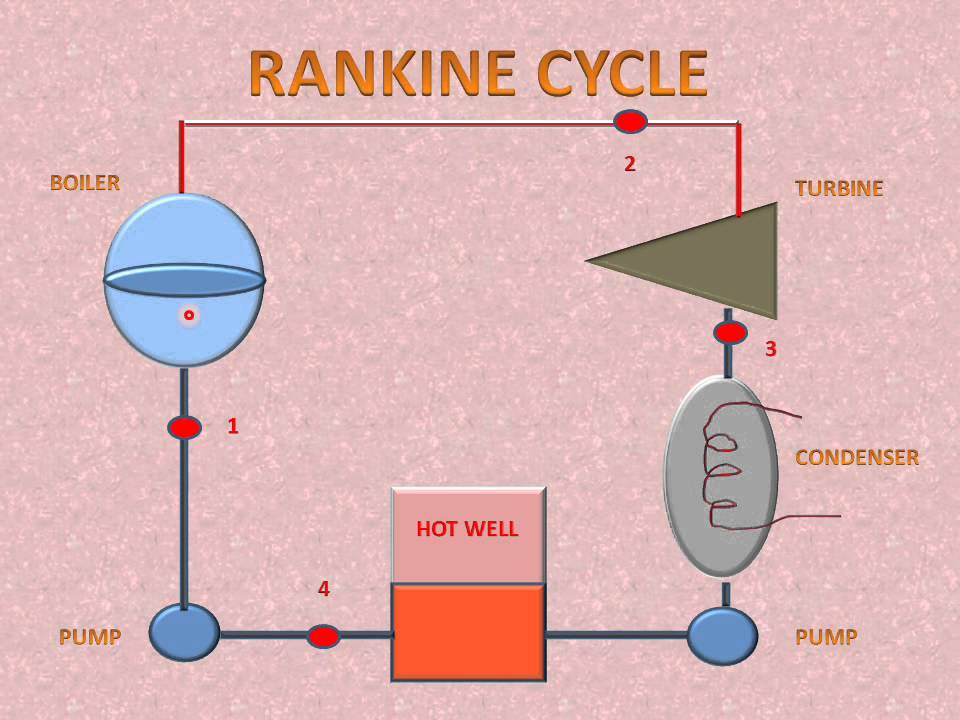## Translate

### Simple Rankine Cycle With T-S DiagramThe simple Rankine cycle is also a ideal vapour cycle. The Rankine Cycle is one of many
thermodynamic cycles that is used to produce mechanical work. This cycle in particular is used to predict the work produced by a steam turbine system in a heat engine. It plays a major role in steam power plants. This cycle is mainly based on the conversion of input heat energy into output power, using turbine. The working fluid at the inlet repeatedly undergoes change of phase and in successive operations, energy is transmitted and output power is produced.

Principle components of Rankine cycle:
The four basic components of Rankine cycle are shown in figure 1 each component in the cycle is regarded as control volume, operating at steady state.

Pump: The liquid condensate leaving the condenser at the state 1 is pumped to the operating pressure of the boiler. The pump operation is considered isentropic.

Boiler: The heat is supplied in the working fluid (feed water) in the boiler and thus vapor is generated. The vapor leaving the boiler is either at saturated at the state 3 or superheated at the state 3'', depending upon the amount of heat supplied by the boiler.

Turbine: The vapor leaving the boiler enters the turbine, where it expands isentropically to the condenser pressure at the state 4. The work produced by the turbine is rotary (shaft) work and is used to drive an electric generator or machine.

Condenser: The condenser is attached at the exit of the turbine. The vapor leaving the turbine is wet vapor and it is condensed completely in the condenser to the state 1, by giving its latest heat to some other cooling fluid like water.

The various processes in simple Rankine cycle are:

1–2: Reversible adiabatic pumping process in the pump,
2–3: Constant-pressure transfer of heat in the boiler,
3–4: Reversible adiabatic expansion in the turbine (or other prime movers such as a steam engine),
4–1: Constant-pressure transfer of heat in the condenser.The Rankine cycle also includes the possibility of superheating the vapor, as cycle 1–2–3'–4'–1.

If kinetic and potential energy changes are neglected, heat transfer and work may be represented by various areas on the T–s diagram. The heat transferred to the working fluid is represented by area
a–2–2'–3–b–a and the heat transferred from the working fluid by area a–1–4–b–a. From the first law of thermodynamics we can conclude that the area representing the work is the difference between these two areas—area 1–2–2'–3–4–1. The thermal efficiency is defined by the relationFor analyzing the Rankine cycle, it is helpful to think of efficiency as depending on the average temperature at which heat is supplied and the average temperature at which heat is rejected. Any changes that increase the average temperature at which heat is supplied or decrease the average temperature heat is rejected will increase the Rankine-cycle efficiency.

In analyzing the ideal cycles in this chapter, the changes in kinetic and potential energies from one point in the cycle to another are neglected. In general, this is a reasonable assumption for the actual cycles.

The Rankine cycle has lower thermal efficiency than a Carnot cycle thermal efficiency with the same maximum and minimum temperatures as a Rankine cycle because of the average temperature between 2 and 2| is less than the temperature during evaporation.

Efficiency of Simple Rankine Cycle:
As we know, efficiency is the ratio between output and input. Here the output is work done and input is heat energy.
Net work done = work done in turbine + work done in pump
Net heat transfer = heat produced in boiler + heat rejected in condenser.